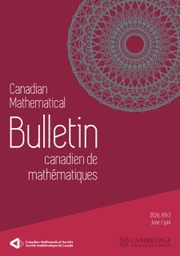Home
Hostname: page-component-7ccbd9845f-w45k2 Total loading time: 0.78 Render date: 2023-01-27T07:22:28.282Z Has data issue: true Feature Flags: { "useRatesEcommerce": false } hasContentIssue falseCanadian Mathematical Bulletin

# Cyclic inner functions in growth classes and applications to approximation problems

Published online by Cambridge University Press:  23 November 2022

## Abstract

It is well known that for any inner function$\theta$ defined in the unit disk$\mathbb {D}$ , the following two conditions: (i) there exists a sequence of polynomials$\{p_n\}_n$ such that$\lim _{n \to \infty } \theta (z) p_n(z) = 1$ for all$z \in \mathbb {D}$ and (ii)$\sup _n \| \theta p_n \|_\infty < \infty$ , are incompatible, i.e., cannot be satisfied simultaneously. However, it is also known that if we relax the second condition to allow for arbitrarily slow growth of the sequence$\{ \theta (z) p_n(z)\}_n$ as$|z| \to 1$ , then condition (i) can be met for some singular inner function. We discuss certain consequences of this fact which are related to the rate of decay of Taylor coefficients and moduli of continuity of functions in model spaces$K_\theta$ . In particular, we establish a variant of a result of Khavinson and Dyakonov on nonexistence of functions with certain smoothness properties in$K_\theta$ , and we show that the classical Aleksandrov theorem on density of continuous functions in$K_\theta$ is essentially optimal. We consider also the same questions in the context of de Branges–Rovnyak spaces$\mathcal {H}(b)$ and show that the corresponding approximation result also is optimal.

Type
Article

## Access options

Get access to the full version of this content by using one of the access options below. (Log in options will check for institutional or personal access. Content may require purchase if you do not have access.)

## References

Aleksandrov, A. B., Invariant subspaces of shift operators. An axiomatic approach . Zap. Nauchn. Sem. Leningrad. Otdel. Mat. Inst. Steklov. (LOMI) 113(1981), no. 264, 726.Google Scholar
Aleman, A. and Malman, B., Density of disk algebra functions in de Branges–Rovnyak spaces . C. R. Math. Acad. Sci. Paris 355(2017), no. 8, 871875.CrossRefGoogle Scholar
Anderson, J. M., Fernandez, J. L., and Shields, A. L., Inner functions and cyclic vectors in the Bloch space . Trans. Amer. Math. Soc. 323(1991), no. 1, 429448.CrossRefGoogle Scholar
Bouya, B., Closed ideals in analytic weighted Lipschitz algebras . Adv. Math. 219(2008), no. 5, 14461468.CrossRefGoogle Scholar
Cima, J., Matheson, A., and Ross, W., The Cauchy transform, Mathematical Surveys and Monographs, 125, American Mathematical Society, Providence, RI, 2006.CrossRefGoogle Scholar
Dyakonov, K. and Khavinson, D., Smooth functions in star-invariant subspaces . Contemp. Math. 393(2006), 59.CrossRefGoogle Scholar
Fricain, E. and Mashreghi, J., The theory of$\mathcal{H}$ (b) spaces . Vol. 1, New Mathematical Monographs, 20, Cambridge University Press, Cambridge, 2016.Google Scholar
Fricain, E. and Mashreghi, J., The theory of$\mathcal{H}$ (b) spaces . Vol. 2, New Mathematical Monographs, 21, Cambridge University Press, Cambridge, 2016.Google Scholar
Garcia, S. R, Mashreghi, J., and Ross, W. T., Introduction to model spaces and their operators, Cambridge University Press, Cambridge, 2016.CrossRefGoogle Scholar
Hartman, P. and Kershner, R., The structure of monotone functions . Amer. J. Math. 59(1937), no. 4, 809822.CrossRefGoogle Scholar
Khrushchev, S. V., The problem of simultaneous approximation and of removal of the singularities of Cauchy type integrals . Tr. Mat. Inst. Steklova 130(1978), 124195.Google Scholar
Korenblum, B., An extension of the Nevanlinna theory . Acta Mathematica 135(1975), 187219.CrossRefGoogle Scholar
Limani, A. and Malman, B., An abstract approach to approximation in spaces of pseudocontinuable functions . Proc. Amer. Math. Soc. 150(2022), no. 6, 25092519.Google Scholar
Ransford, T., On the decay of singular inner functions . Can. Math. Bull. 64(2021), no. 4, 902905.CrossRefGoogle Scholar
Roberts, J., Cyclic inner functions in the Bergman spaces and weak outer functions in${H}^p$ ,$0<p<1$ . Ill. J. Math. 29(1985), no. 1, 2538.Google Scholar
Sarason, D., Sub-hardy Hilbert spaces in the unit disk, University of Arkansas Lecture Notes in the Mathematical Sciences, 10, John Wiley & Sons, Inc., New York, 1994.Google Scholar
Shapiro, H. S., Weakly invertible elements in certain function spaces, and generators in${\ell}_1$ . Michigan Math. J. 11(1964), no. 1, 161165.CrossRefGoogle Scholar
Shapiro, H. S., A class of singular functions . Can. J. Math. 20(1968), 14251431.CrossRefGoogle Scholar
Tamrazov, P. M., Contour and solid structure properties of holomorphic functions of a complex variable . Russ. Math. Surv. 28(1973), no. 1, 141.CrossRefGoogle Scholar

# Save article to Kindle

Note you can select to save to either the @free.kindle.com or @kindle.com variations. ‘@free.kindle.com’ emails are free but can only be saved to your device when it is connected to wi-fi. ‘@kindle.com’ emails can be delivered even when you are not connected to wi-fi, but note that service fees apply.

Find out more about the Kindle Personal Document Service.

Cyclic inner functions in growth classes and applications to approximation problems
Available formats
×

# Save article to Dropbox

To save this article to your Dropbox account, please select one or more formats and confirm that you agree to abide by our usage policies. If this is the first time you used this feature, you will be asked to authorise Cambridge Core to connect with your Dropbox account. Find out more about saving content to Dropbox.

Cyclic inner functions in growth classes and applications to approximation problems
Available formats
×

# Save article to Google Drive

To save this article to your Google Drive account, please select one or more formats and confirm that you agree to abide by our usage policies. If this is the first time you used this feature, you will be asked to authorise Cambridge Core to connect with your Google Drive account. Find out more about saving content to Google Drive.

Cyclic inner functions in growth classes and applications to approximation problems
Available formats
×
×